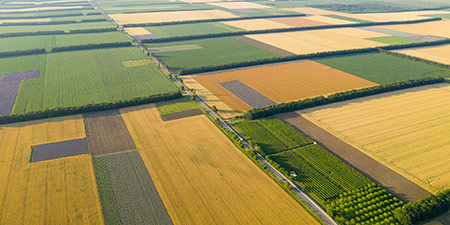# Square Foot (ft²) to Square Yard (yd²)

Area Units ﹣ Converter Square Foot to Square Yard

Here you can convert the Area unit Square Foot into the unit Square Yard and vice versa you can convert Square Yard into Square Foot. By clicking the "Swap units" icon, you will always obtain the desired conversion in the calculation result, i.e., ft² to yd² or yd² to ft². With the following calculator you can also calculate any other Area unit.

A square foot (ft²; plural square feet) is equal to the area of a square with a side length of one foot (30.48 cm).

1 ft² = 1 ft × 1 ft

1 ft² = 30,48 cm × 30,48 cm

A foot is 12 inches long in the Anglo-American system and thus a square foot has 144 square inches. In the Anglo-American world, the size of land is indicated in land and property surveys using only the two area units, acre and square foot.

A square yard (yd²) is equal to the area of a square with a side length of one yard (0.9144 meters).

1 yd² = 1 yd × 1 yd

1 yd² = 0,9144 m × 0,9144 m

## Basis for conversion Square Foot (ft²) to Square Yard (yd²) and vice versa

The abbreviation for the "Area unit Square Foot" is ft². The abbreviation for the "Area unit Square Yard" is yd².

## Formula for the conversion of Square Foot (ft²) to Square Yard (yd²) and vice versa

The calculation from Square Foot to Square Yard shall be made using the following conversion formula:

Conversion formula Square Foot to Square Yard

Determine the number of Square Yard from Square Foot

Square Foot × 0.11111111111111

## Formula for the conversion of Square Yard (yd²) to Square Foot (ft²)

The calculation from Square Yard to Square Foot shall be made using the following conversion formula:

Conversion formula Square Yard to Square Foot

Determine the number of Square Foot from Square Yard

Square Yard × 9

## Overview table : How many Square Foot are in a Square Yard ?

Square Foot ft² ⇒ Square Yard yd²
0.01 ft²  are  0.00111 yd²
0.02 ft²  are  0.00222 yd²
0.03 ft²  are  0.00333 yd²
0.04 ft²  are  0.00444 yd²
0.05 ft²  are  0.00555 yd²
0.06 ft²  are  0.00666 yd²
0.07 ft²  are  0.00777 yd²
0.08 ft²  are  0.00888 yd²
0.09 ft²  are  0.01000 yd²
0.10 ft²  are  0.01111 yd²
0.20 ft²  are  0.02222 yd²
0.30 ft²  are  0.03333 yd²
0.40 ft²  are  0.04444 yd²
0.50 ft²  are  0.05555 yd²
0.60 ft²  are  0.06666 yd²
0.70 ft²  are  0.07777 yd²
0.80 ft²  are  0.08888 yd²
0.90 ft²  are  0.10000 yd²
1 ft²  corresponds to  0.11111 yd²
2 ft²  are  0.22222 yd²
3 ft²  are  0.33333 yd²
4 ft²  are  0.44444 yd²
5 ft²  are  0.55555 yd²
6 ft²  are  0.66666 yd²
7 ft²  are  0.77777 yd²
8 ft²  are  0.88888 yd²
9 ft²  are  1 yd²
10 ft²  are  1.11111 yd²
20 ft²  are  2.22222 yd²
30 ft²  are  3.33333 yd²
40 ft²  are  4.44444 yd²
50 ft²  are  5.55555 yd²
60 ft²  are  6.66666 yd²
70 ft²  are  7.77777 yd²
80 ft²  are  8.88888 yd²
90 ft²  are  10 yd²
100 ft²  are  11.11111 yd²
200 ft²  are  22.22222 yd²
300 ft²  are  33.33333 yd²
400 ft²  are  44.44444 yd²
500 ft²  are  55.55555 yd²
600 ft²  are  66.66666 yd²
700 ft²  are  77.77777 yd²
800 ft²  are  88.88888 yd²
900 ft²  are  100 yd²
1 000 ft²  are  111.11111 yd²

## Overview table : How many Square Yard are in a Square Foot ?

Square Yard yd² ⇒ Square Foot ft²
0.01 yd²  are  0.09000 ft²
0.02 yd²  are  0.18000 ft²
0.03 yd²  are  0.27000 ft²
0.04 yd²  are  0.36000 ft²
0.05 yd²  are  0.45000 ft²
0.06 yd²  are  0.54000 ft²
0.07 yd²  are  0.63000 ft²
0.08 yd²  are  0.72000 ft²
0.09 yd²  are  0.81000 ft²
0.10 yd²  are  0.90000 ft²
0.20 yd²  are  1.80000 ft²
0.30 yd²  are  2.70000 ft²
0.40 yd²  are  3.60000 ft²
0.50 yd²  are  4.50000 ft²
0.60 yd²  are  5.40000 ft²
0.70 yd²  are  6.30000 ft²
0.80 yd²  are  7.20000 ft²
0.90 yd²  are  8.10000 ft²
1 yd²  corresponds to  9 ft²
2 yd²  are  18 ft²
3 yd²  are  27 ft²
4 yd²  are  36 ft²
5 yd²  are  45 ft²
6 yd²  are  54 ft²
7 yd²  are  63 ft²
8 yd²  are  72 ft²
9 yd²  are  81 ft²
10 yd²  are  90 ft²
20 yd²  are  180 ft²
30 yd²  are  270 ft²
40 yd²  are  360 ft²
50 yd²  are  450 ft²
60 yd²  are  540 ft²
70 yd²  are  630 ft²
80 yd²  are  720 ft²
90 yd²  are  810 ft²
100 yd²  are  900 ft²
200 yd²  are  1 800 ft²
300 yd²  are  2 700 ft²
400 yd²  are  3 600 ft²
500 yd²  are  4 500 ft²
600 yd²  are  5 400 ft²
700 yd²  are  6 300 ft²
800 yd²  are  7 200 ft²
900 yd²  are  8 100 ft²
1 000 yd²  are  9 000 ft²

## Source information

As source for the information in the 'Area Units' category, we have used in particular:

## Last update

This page of the 'Area Units' category was last edited or reviewed by Michael Mühl on February 20, 2023. It corresponds to the current status.

### Changes in this category "Area Units"

• Publication of Area Converter together with associated texts.